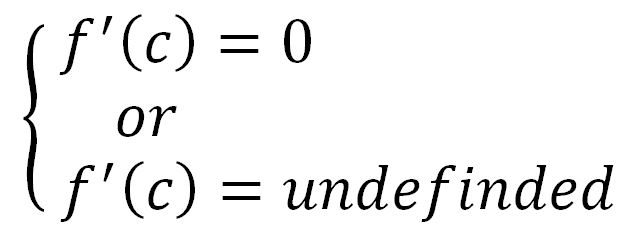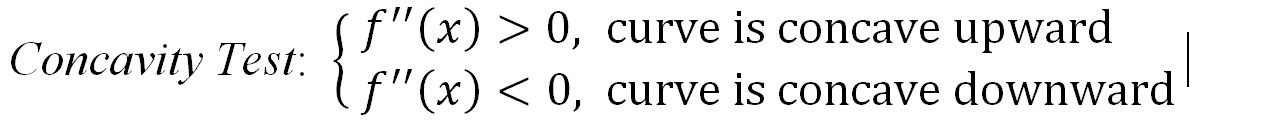# Curve sketching

### Curve sketching

In this section we will expand our knowledge on the connection between derivatives and the shape of a graph. By following the "5-Steps Approach", we will quantify the characteristics of the function with application of derivatives, which will enable us to sketch the graph of a function.

#### Lessons

Guidelines for Curve Sketching
a) domain

b) Intercepts
y-intercept: set x=0 and evaluate y.
x-intercept: set y=0 and solve for x. (skip this step if the equation is difficult to solve)

c) Asymptotes
vertical asymptotes:
for rational functions, vertical asymptotes can be located by equating the denominator to 0 after canceling any common factors.

horizontal asymptotes:
evaluate $lim_{x \to \infty } f(x)$ to determine the right-end behavior; evaluate $lim_{x \to -\infty } f(x)$ to determine the left-end behavior.

slant asymptotes:
for rational functions, slant asymptotes occur when the degree of the numerator is one more than the degree of the denominator.

d) Compute$f' (x)$
find the critical numbers:• use the First Derivative Test to find: intervals of increase/decrease and local extrema.

e) Compute$f'' (x)$
• inflection points occur where the direction of concavity changes. find possible inflection points by equating the$f'' (x)$ to 0.

Concavity Test:inflection points occur where the direction of concavity changes.
• 1.
Use the guidelines to sketch the graph of:

$f(x)=\frac{x^3-8}{x^3+8}$
a)
Domain

b)
Intercepts

c)
Asymptotes

d)
Compute $f' (x):$
- critical numbers
- intervals of increase/decrease
- local extrema

e)
Compute $f'' (x):$
- possible inflection points
- intervals of concavity
- verify inflection points

• 2.
Use the guidelines to sketch the graph of:
$f(x)=-x^3-6x^2-9x$
a)
Domain

b)
Intercepts

c)
Asymptotes

d)
Compute $f' (x):$
- critical numbers
- intervals of increase/decrease
- local extrema

e)
Compute $f'' (x):$
- possible inflection points
- intervals of concavity
- verify inflection points